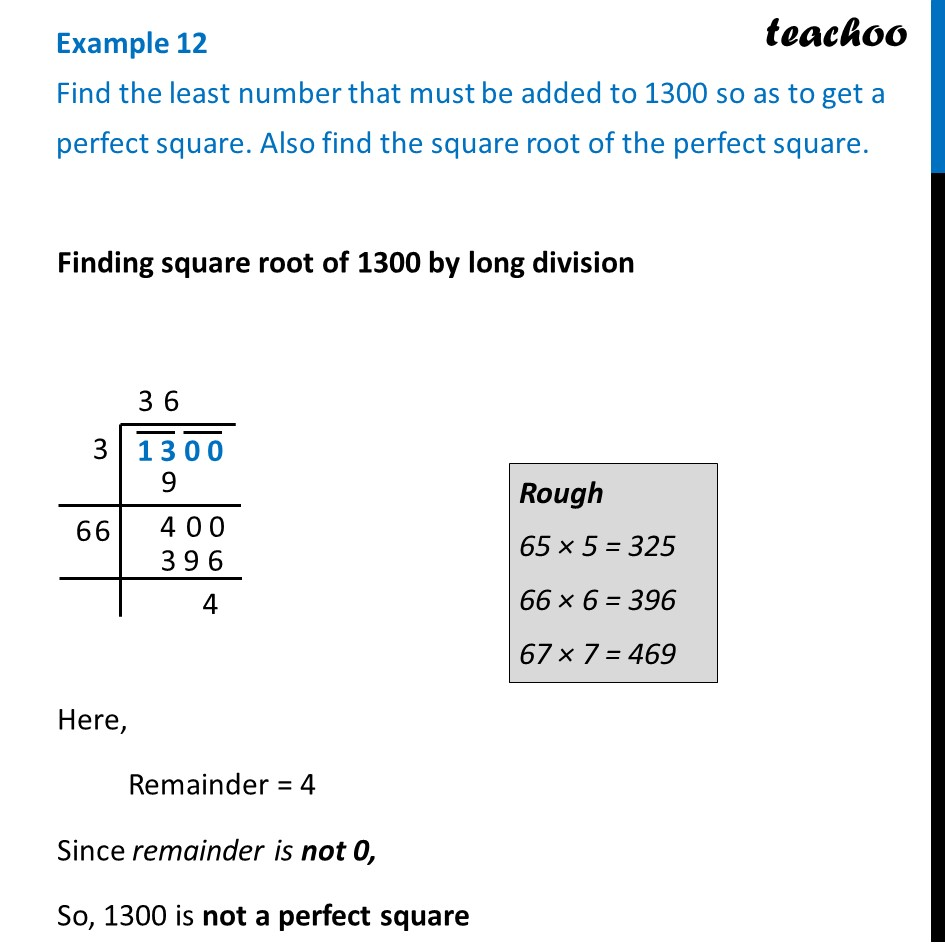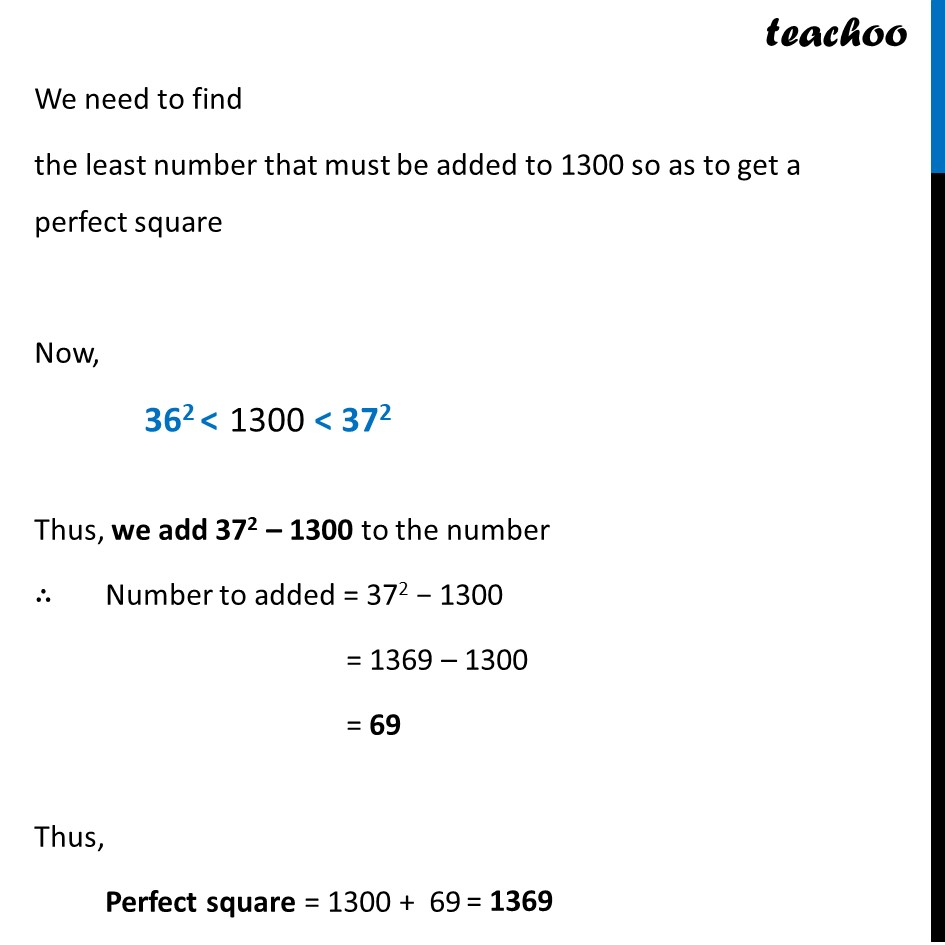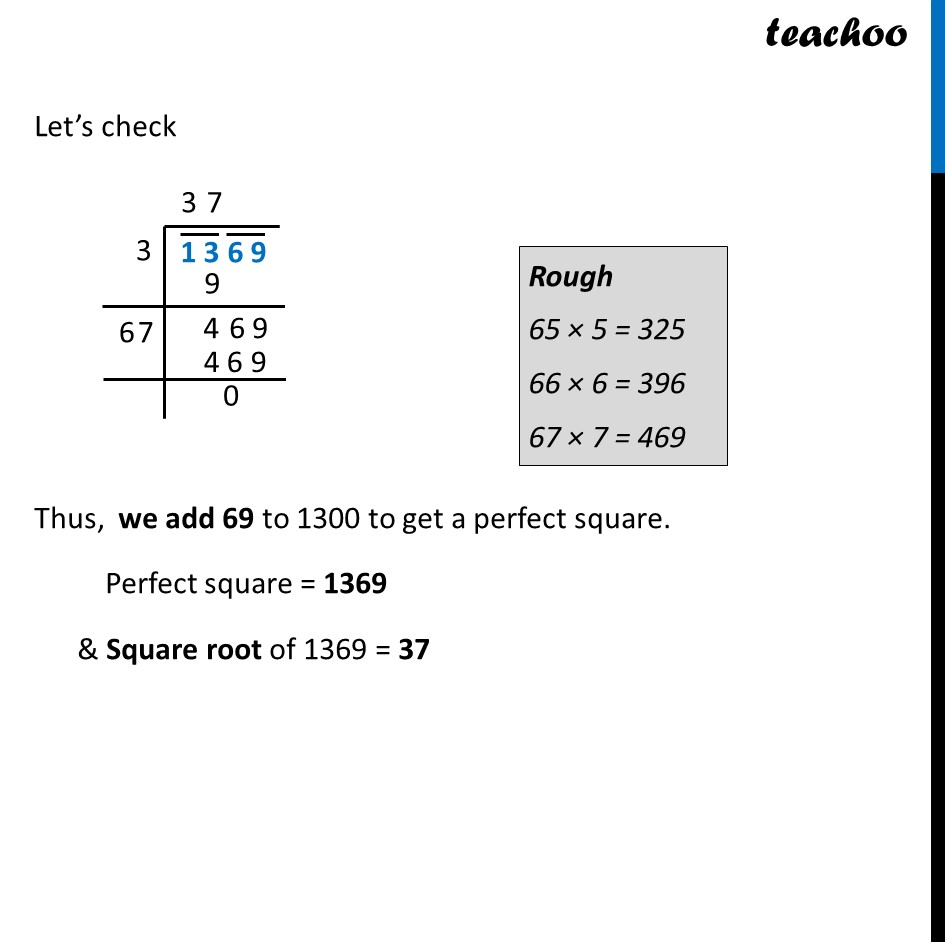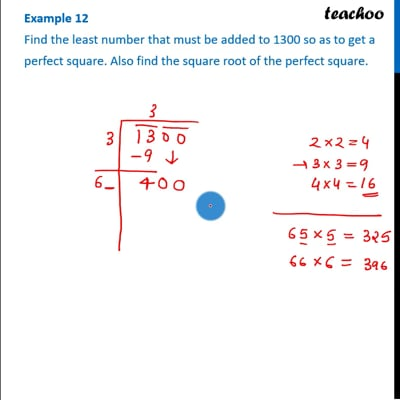Examples

Chapter 6 Class 8 Squares and Square Roots
Serial order wiseThis video is only available for Teachoo black users

### Transcript

Example 12 Find the least number that must be added to 1300 so as to get a perfect square. Also find the square root of the perfect square. Finding square root of 1300 by long division Finding square root of 1300 by long division Here, Remainder = 4 Since remainder is not 0, So, 1300 is not a perfect square We need to find the least number that must be added to 1300 so as to get a perfect square Now, Thus, we add 372 – 1300 to the number ∴ Number to added = 372 − 1300 = 1369 – 1300 = 69 Thus, Perfect square = 1300 + 69 Let’s check Thus, we add 69 to 1300 to get a perfect square. Perfect square = 1369 & Square root of 1369 = 37